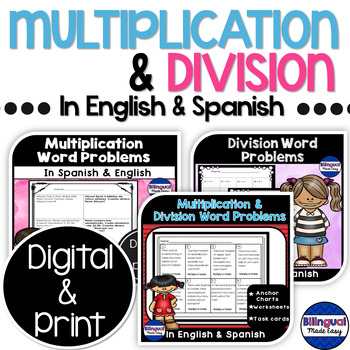# Bilingual Multiplication and Division Word Problems Bundle in English & SpanishSubject
Resource Type
File Type

Zip

(15 MB|56 pages)
Product Rating
4.0
(2 Ratings)
Standards
2 Products in this Bundle
2 products
1. Do your English Language Learners need help solving multiplication word problems? This fabulous variety of bilingual math word problems worksheets helps students learn this mathematical concept. No need to translate word problems these no prep worksheets do the job for you.
2. Do your English Language Learners need help with division word problems? This fabulous variety of Spanish and English division word problems can help. No need to translate word problems because these bilingual no prep worksheets do the work for you.
Also included in:
1. Bilingual Math Mega Bundle contains 6 different math concepts. Each product contains worksheets that are both in English and Spanish. With these all of your English Language Learners can work together within the same lesson.
\$39.97
\$36.00
Save \$3.97
2. SAVE \$\$ by purchasing 4 Math Units for a discounted price. The Third Grade Bilingual Math bundle are products meant to compliment your Bilingual or ESL curriculum. Each worksheet has an identical worksheet in English and Spanish. Meet all of your English Language Learners needs by having these wor
\$38.99
\$38.00
Save \$0.99
3. Everything you need to teach the Third Grade Math Common Core curriculum are included in this bundle. Save \$\$\$ by purchasing all 19 products for one low price. Included are no - prep worksheets to teach multiplication, division, fractions, rounding, and addition with regrouping. Also included are
\$129.94
\$100.00
Save \$29.94
4. Everything you need to teach the Third Grade Math Common Core curriculum are included in this bundle. Save \$\$\$ by purchasing all 18 products for one low price. Included are no - prep worksheets to teach multiplication, division, fractions, rounding, and addition with regrouping. Also included are
\$139.94
\$100.00
Save \$39.94
5. The Multiplication Word Problems no prep worksheets are a great introduction to an essential third and fourth grade mathematics skill. Once my students have mastered understanding Multiplication word problems, I introduce the Division word problems worksheets followed by the Multiplication and Divi
\$15.99
\$12.00
Save \$3.99
6. The Multiplication Word Problems no prep worksheets are a great introduction to an essential third and fourth grade mathematics skill. Once my students have mastered understanding Multiplication word problems, I introduce the Division word problems worksheets followed by the Multiplication and Divi
\$15.99
\$12.00
Save \$3.99
• Bundle Description
• StandardsNEW

Bilingual Multiplication and Division Word Problems Bundle are two products that focus on word problems and computation. Each worksheet is in English and Spanish so that all of your English Language Learners can work at the same time. This bundle was created as a resource that you can use in your Bilingual or ESL classroom.

Also included in Bilingual Multiplication & Division Mega Packs

Make sense of problems and persevere in solving them. Mathematically proficient students start by explaining to themselves the meaning of a problem and looking for entry points to its solution. They analyze givens, constraints, relationships, and goals. They make conjectures about the form and meaning of the solution and plan a solution pathway rather than simply jumping into a solution attempt. They consider analogous problems, and try special cases and simpler forms of the original problem in order to gain insight into its solution. They monitor and evaluate their progress and change course if necessary. Older students might, depending on the context of the problem, transform algebraic expressions or change the viewing window on their graphing calculator to get the information they need. Mathematically proficient students can explain correspondences between equations, verbal descriptions, tables, and graphs or draw diagrams of important features and relationships, graph data, and search for regularity or trends. Younger students might rely on using concrete objects or pictures to help conceptualize and solve a problem. Mathematically proficient students check their answers to problems using a different method, and they continually ask themselves, "Does this make sense?" They can understand the approaches of others to solving complex problems and identify correspondences between different approaches.
Identify arithmetic patterns (including patterns in the addition table or multiplication table), and explain them using properties of operations. For example, observe that 4 times a number is always even, and explain why 4 times a number can be decomposed into two equal addends.
Fluently multiply and divide within 100, using strategies such as the relationship between multiplication and division (e.g., knowing that 8 × 5 = 40, one knows 40 ÷ 5 = 8) or properties of operations. By the end of Grade 3, know from memory all products of two one-digit numbers.
Apply properties of operations as strategies to multiply and divide. Examples: If 6 × 4 = 24 is known, then 4 × 6 = 24 is also known. (Commutative property of multiplication.) 3 × 5 × 2 can be found by 3 × 5 = 15, then 15 × 2 = 30, or by 5 × 2 = 10, then 3 × 10 = 30. (Associative property of multiplication.) Knowing that 8 × 5 = 40 and 8 × 2 = 16, one can find 8 × 7 as 8 × (5 + 2) = (8 × 5) + (8 × 2) = 40 + 16 = 56. (Distributive property.)
Use multiplication and division within 100 to solve word problems in situations involving equal groups, arrays, and measurement quantities, e.g., by using drawings and equations with a symbol for the unknown number to represent the problem.
Total Pages
56 pages
N/A
Teaching Duration
1 month
Report this Resource to TpT
Reported resources will be reviewed by our team. Report this resource to let us know if this resource violates TpT’s content guidelines.